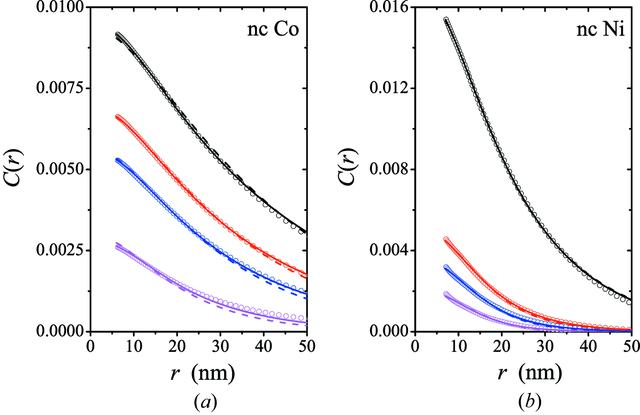disable zoom     view article Figure 14 Comparison between experimental and theoretical data. (Open circles) Correlation functions of the spin-misalignment SANS cross section of (a) nanocrystalline Co and (b) nanocrystalline Ni with average crystallite sizes of D = 10 nm (Co) and D = 49 nm (Ni) (Weissmüller et al., 2001). C(r) data are taken from Michels et al. (2003). Solid lines: fit based on equation (36); dashed lines: fit based on equation (41). Values of the internal magnetic field Hi (in mT) from top to bottom, respectively: (a) 54, 80, 107, 243; (b) 190, 570, 800, 1240. In both analyses, we have used the sphere form factor for P(q) and S(q) = 1.JOURNAL OFAPPLIEDCRYSTALLOGRAPHY
ISSN: 1600-5767
Volume 48| Part 5| October 2015| Pages 1437-1450